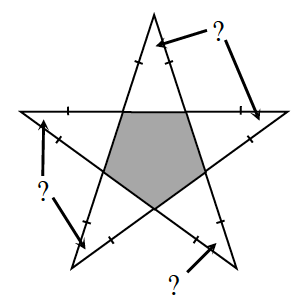### Home > INT2 > Chapter 10 > Lesson 10.1.3 > Problem10-35

10-35.After doing well on a test, Althea’s teacher places a gold star on her paper. When Althea examines the star closely, she realizes that it is really a regular pentagon surrounded by five isosceles triangles, as shown in the diagram at right. What is the measure of each vertex angle of the star? Show all work.

What is the measure of each interior angle of a regular pentagon if its total angle sum is $540°$?

Once you have the measures of the pentagon, how can you use these
measures to find the measures of the angles in the isosceles triangles?

Use the Triangle Angle Sum Theorem to find the vertex angle of each star point.